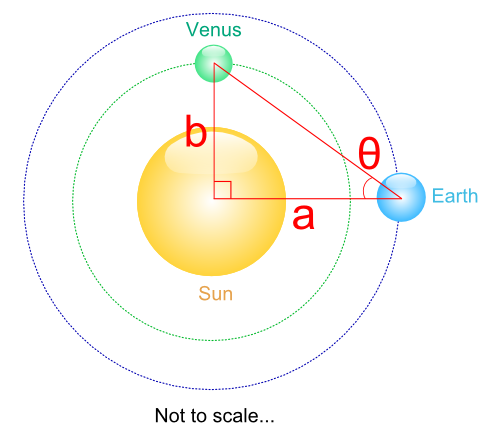#### You may also like### Golden Thoughts

Rectangle PQRS has X and Y on the edges. Triangles PQY, YRX and XSP have equal areas. Prove X and Y divide the sides of PQRS in the golden ratio.### At a Glance

The area of a regular pentagon looks about twice as a big as the pentangle star drawn within it. Is it?### Contact

A circular plate rolls in contact with the sides of a rectangular tray. How much of its circumference comes into contact with the sides of the tray when it rolls around one circuit?

# A Scale for the Solar System

##### Age 14 to 16Challenge Level

Lukaz Smith, from Parkside School, sent in the following correct approach to finding the ratio of the Earth-Sun and Venus-Sun distances (diagram added to help clarify the solution)

If you said the Earth to sun distance was 1 astronomical unit ($a$ in diagram), then you could simply measure the angle ($\theta$ in diagram) between Sun, Earth and Venus, when you knew that there was a 90 degree angle between Earth, Sun and Venus. Then you just find the tangent of the angle, so it's 1 : $\tan \theta$ as the ratio of Earth to Sun distance and Venus to Sun distance.